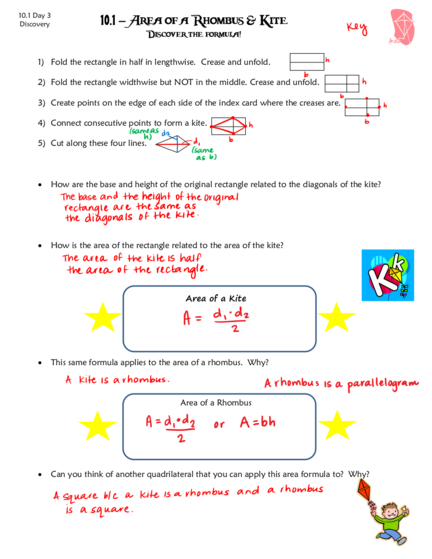# Area of a Rhombus and KiteContributed by:This pdf is based on finding the areas of rhombus and kites. The area of a kite is half the product of the lengths of its diagonals.
Area of a rhombus or any parallelogram = base × height.
1. 10.1 Day 3
Discovery 10.1 – Area of a Rhombus & Kite
Discover the formula!
1) Fold the rectangle in half in lengthwise. Crease and unfold.
2) Fold the rectangle widthwise but NOT in the middle. Crease and unfold.
3) Create points on the edge of each side of the index card where the creases are.
4) Connect consecutive points to form a kite.
5) Cut along these four lines.
 How are the base and height of the original rectangle related to the diagonals of the kite?
 How is the area of the rectangle related to the area of the kite?
Area of a Kite
 This same formula applies to the area of a rhombus. Why?
Area of a Rhombus
 Can you think of another quadrilateral that you can apply this area formula to? Why?
2. Let’s try a few examples:
1. Find d2 of a kite in which d1 = 16 cm 2. Find the area of a rhombus with
and A = 48 cm2. d1 = (6x + 4) in. and d2 = (10x + 10) in.
3. Find the area of the kite below: 4. Find the area of the rhombus below:
d1 = 24
6 d2 = 12
24
26
5. Find the area of a rhombus whose perimeter 6. Find the area of the kite given KIT is a
is 20 and whose longer diagonal is 8. Right angle , KT = 8 and KE = 5.
(Hint: Draw a picture to help you!) (Picture is not drawn to scale!)
K
I E
T